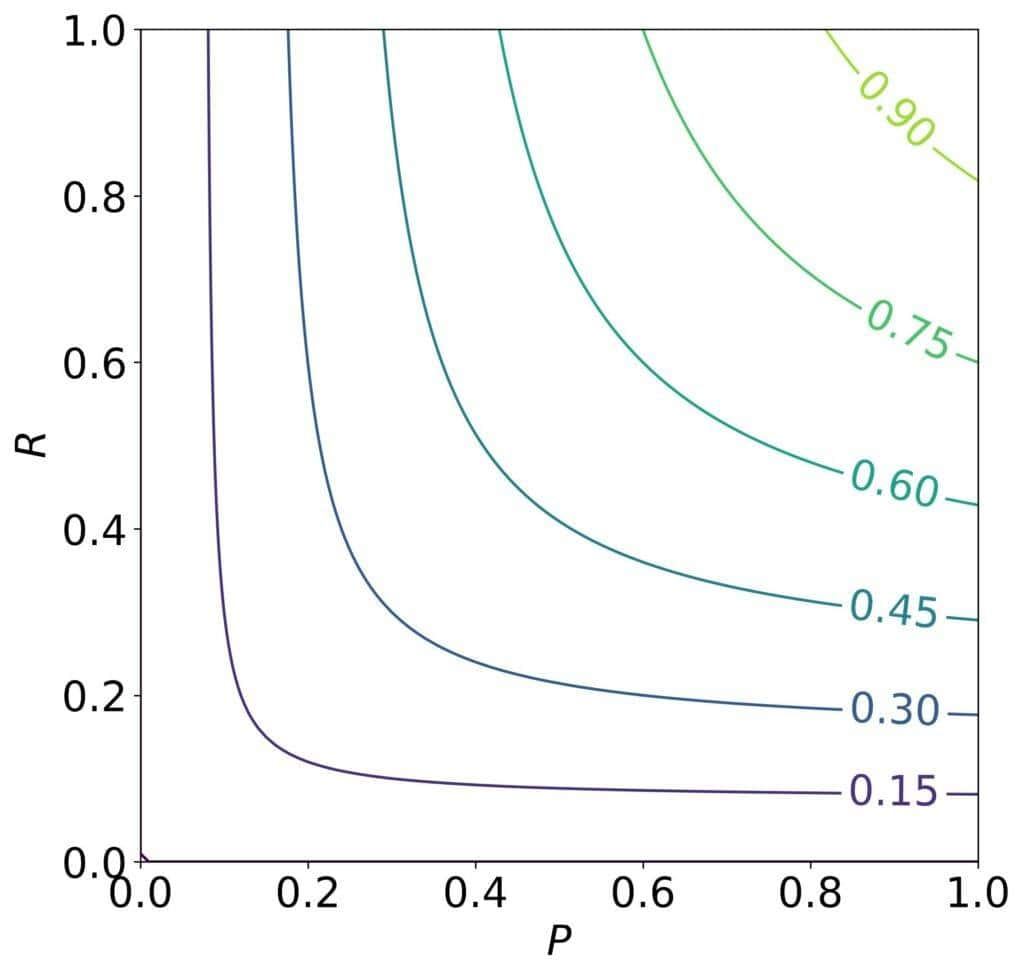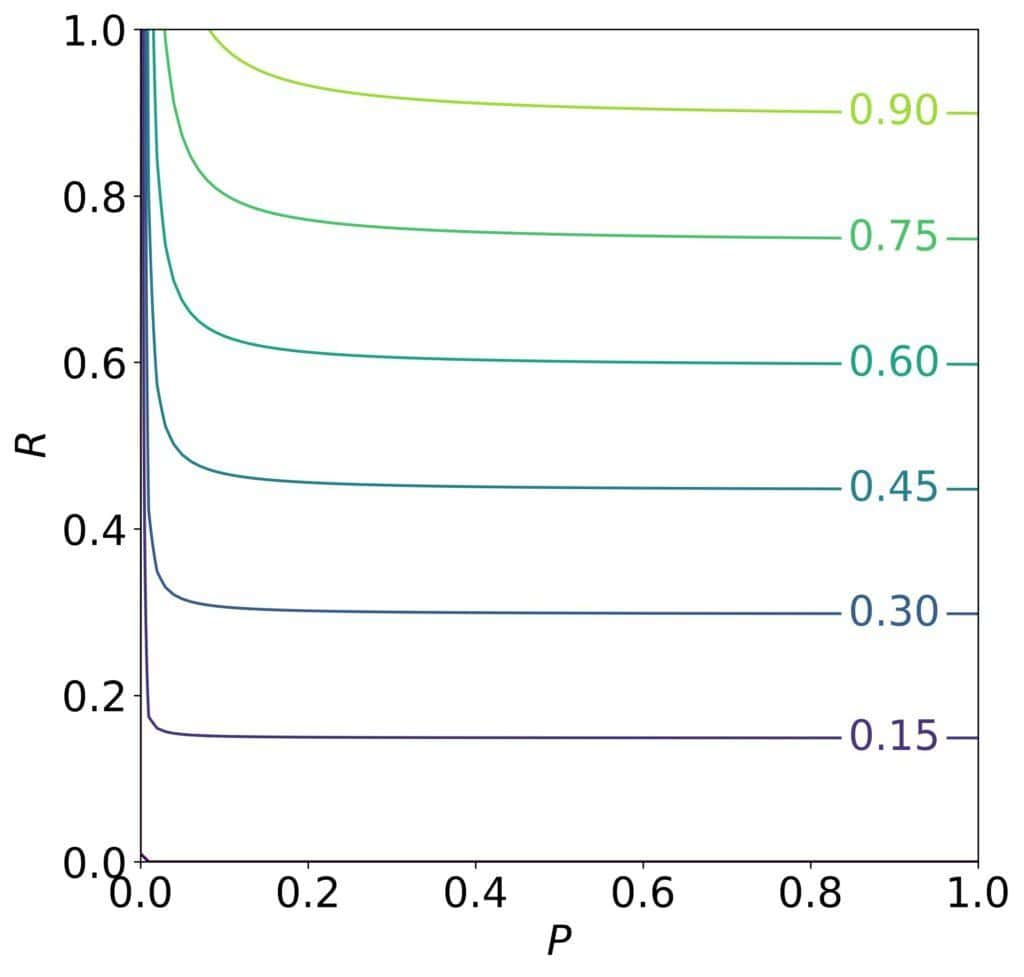## 1. Introduction

There are several metrics for evaluating machine-learning (ML) models. One that we often calculate when analyzing classifiers is thescore, which combines precision and recall into a single value.

In this tutorial, we’ll talk about its generalization, thescore, which can give more weight to either recall or precision.

## 2. The F1 Score

The F1 score of a classifier is the harmonic mean of its precisionand recall:

(1)It’s useful because it’s high when both scores are large, as we see in its contour plot:It gives equal weights to recall and precision, so the contours are symmetric around the 45-degree line,

### 2.1. What if Precision and Recall Aren’t Equally Important?

However, there are cases where one of the scores is more important than the other.

We care more about recall if a false negative is more severe an error than a false positive. Automated diagnostic ML tools in medicine illustrate that. There, a false negative is a missed condition, which could be fatal for our patient’s health. In contrast, a false-positive diagnosis induces stress, but additional testing can relieve the patient.

Conversely, precision is more important when a false positive has a higher cost. That’s the case in spam detection. Letting a spam e-mail appear in the inbox may annoy the user, but marking a non-spam e-mail as spam and sending it to thrash could result in the loss of a job opportunity.

In such applications, we’d like to have a metric that considers the relative importance ofand. Thescore does precisely that.

## 3. The F-Beta Score

The common formulation ofis:

(2)It’s a weighted harmonic mean ofandwhich usesandas the weights:

(3)If, the recall istimes more important than precision, and if, it’s the other way around. As the contours forshow, we can get a highscore if the recall is high enough no matter if the precision is low, which aligns with our requirements:But why doesfigure in the equations instead of? Isn’t the latter more intuitive?

### 3.1. Relative Importance of Precision and Recall

The reason why we haveinstead oflies in how the relative importance was defined whenwas first formulated.

In general, the weighted harmonic mean ofandusingandas the weights is:

(4)To getfrom, we require the latter to satisfy the condition of relative importance. More precisely, we wantto be such that at the points at whichandequally contribute to,istimes.

Mathematically, that means that the ratioshould be equal towhen the partial derivativesandare the same.

### 3.2. Derivation

Let’s first find the derivatives:

(5)From, we get:

(6)Requiring the ratioto be, we solvefor:

(7)Plugging ininto the weighted harmonic mean, we getas defined by Equations (2) and (3).

### 3.3. The Effect of Importance

Let’s analyze what happens toas we vary.

Settingto 1, we get the usual. That covers the case withandhaving equal weights.

If only recall is important, we let. In that case, we expectto reduce to. Taking the limit, we get:

(8)Similarly, if we care only about precision, we setto 0:

(9)The values ofbetween 0 andrepresent intermediate cases.

## 4. Alternative Formulation of the F-Beta Score

A different definition of relative importance would yield a differentscore.

For instance, we could say that if we consider the recall score to betimes more important than precision, that means that when, increasingimprovestimes as much as an equal increase in.

Mathematically, this translates to the following condition:

(10)Solving for, we get:

(11)From there, we get a metric that is linear in:

(12)It too reduces towhenbut uses a different definition of relative importance than the version with.

## 5. Conclusion

In this article, we talked about thescore. We use it to evaluate classifiers when the recall and precision aren’t equally important. For instance, that’s the case in spam detection and medicine.

However, the two scores’ relative importance we quantify withhas a formal mathematical definition: when their partial derivatives are equal, recall istimes as large as precision.